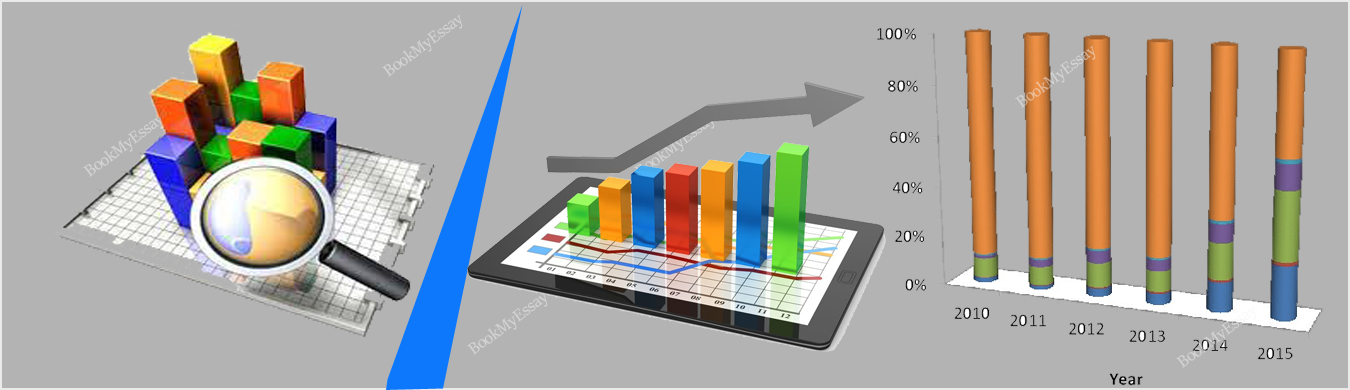Before you can begin to know the facts about the statistics you have to understand about the mean, median and mode. These are three main methods that are used to calculate the best and appropriate numbers. We have to know about the perfect and appropriate technique to use these three methods so that we get the 100% quality result later. To guide the students with best data, we are offering the 100% quality information through assignment writing help on statistics. The main motto is to provide the topic related and best information to students so that they collect the main facts about every topic from our best writers.• The Mean: When we talk about the average in the statistics that means we are referring the mean. Because mean is one of the main and perfect method that provides the appropriate average value according to the numbers. To get the result in mean
1. Simply add all of your selected numbers
2. Divide the sum according to numbers (That you are adding).
3. Then you will get the result. This is one of the simplest methods to get the result by using the mean. This is one of the main and most commonly used method that always give the perfect and assumed result according to the data list. We mainly use this method in the large group of numbers so that we collect the appropriate and quality result.
• The Median: This is the middle value in a data set. To get the number, you have to calculate it, place all the numbers n increasing order. If you have an integer in your number list then in next step s to find the middle number on your selected list. Here we are trying to define the concept through the numbers like:  If you have even number in your selected list, calculate the median requires another step. To get the result, you have to find the two middle integers from your list and add them then divide the number by two. This is the best way to get the result. To get the additional data according to topic, you can easily visit on our website and pick the best options in Statistics assignment writing help service.
• The Mode: In the Statistics, we can easily define the mode as a list of numbers that completely refers to the integers that occurs most frequently. It is different from the mean and median and we can simply get the number through mode about the frequency. That means how many times numbers occurs on the basis of its occurrence. The highest occurrence if the number is answer itself. We get the numbers on the basis if the repetition in the number list. This is one of the simplest and easiest method among the three of them and it is mainly used in the statistics to get the result.

Our online assignment writer offering the dynamic Statistics assignment help in Australia which were distributed to the students all over the world. These assignments are the entire package that covers homework, inquiry, writing surveys.